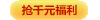全栈开发培训之值得深思的面试问题

10个问题，看似简单，但是每个背后都涵盖了一个或几个大家容易忽视的基础知识点，希望能够帮助到你的面试和平时工作。

Q1第一个问题关于 弱类型

1. <?php
3. \$str2 = 'yaba';
4. if (strpos(\$str1,\$str2)) {
5.     echo "\"" . \$str1 . "\" contains \"" . \$str2 . "\"";
6. } else {
7.     echo "\"" . \$str1 . "\" does not contain \"" . \$str2 . "\"";
8. }

strpos 是返回字符串 str2 在 str1 的位置，没有找到则返回 false ，然而实际上这次返回了 0 而在if语句中0也被当作false,所以我们需要对false做类型判断，正确的代码如下:

1. <?php
3.       \$str2 = 'yaba';
4.       if (strpos(\$str1,\$str2) !== false) {
5.           echo "\"" . \$str1 . "\" contains \"" . \$str2 . "\"";
6.       } else {
7.             echo "\"" . \$str1 . "\" does not contain \"" . \$str2 . "\"";
8.       }

Q2 ：下面的输出结果会是怎样？

1. <?php
2.         \$x = 5;
3.         echo \$x;
4.        echo "<br />";
5.        echo \$x+++\$x++;
6.        echo "<br />";
7.        echo \$x;
8.        echo "<br />";
9.        echo \$x---\$x--;
10.        echo "<br />";
11.         echo \$x;

Q3：关于变量的引用；

1. <?php
2.       \$a = '1';
3.       \$b = &\$a;
4.       \$b = "2\$b";

Q4：下面是true还是false
1. <font size="2"><?php
2. var_dump(0123 == 123);
3. var_dump('0123' == 123);
4. var_dump('0123' === 123);</font>

var_dump(0123 == 123);// false ,PHP会默认把0123当作8进制来处理，实际转化为10进制就是83，显然这不是相等的。
var_dump('0123' == 123);// true 这里php会非常有趣的将'0123'转换成一个数字而且默认去掉了前面的0也就是 123==123
var_dump('0123' === 123);// false 很显然上面的问题已经说过了数字和字符串类型不一致。

Q5：下面的代码有什么问题吗？输出会是什么，怎样修复它
1. <font size="2"><?php
2.        \$referenceTable = array();
3.        \$referenceTable['val1'] = array(1, 2);
4.        \$referenceTable['val2'] = 3;
5.        \$referenceTable['val3'] = array(4, 5);
6.        \$testArray = array();
7.         \$testArray = array_merge(\$testArray, \$referenceTable['val1']);
8.        var_dump(\$testArray);
9.        \$testArray = array_merge(\$testArray, \$referenceTable['val2']);
10.        var_dump(\$testArray);
11.       \$testArray = array_merge(\$testArray, \$referenceTable['val3']);
12.       var_dump(\$testArray);  </font>

array(2) { => int(1) => int(2) }
NULL
NULL

Warning: array_merge(): Argument #2 is not an array
Warning: array_merge(): Argument #1 is not an array

array_merge 需要传入的参数都是数组，如果不是，则会返回null。 你可以这样修改

1. <?php
2. \$testArray = array_merge(\$testArray, (array)\$referenceTable['val1']);
3. var_dump(\$testArray);
4. \$testArray = array_merge(\$testArray, (array)\$referenceTable['val2']);
5. var_dump(\$testArray);
6. \$testArray = array_merge(\$testArray, (array)\$referenceTable['val3']);
7. var_dump(\$testArray);

Q6: \$x应该是输出什么？
1. <?php
2.         \$x = true and false;
3.         var_dump(\$x);  </font>

\$x = true;
true and false

Q7:经过下面的运算 \$x的值应该是多少？
1. \$x = 3 + "15%" + "\$25"

1. \$x = 3 + 15 + 0

Q8:运行下面的代码， \$text 的值是多少？ strlen(\$text) 又会返回什么结果？

<？php
\$text = 'John ';
\$text = 'Doe';

\$text = "Doe" 给某个字符串具体的某个位置具体字符时候，实际只会把D赋给 \$text . 虽然 \$text 才开始只有5个自负长度，但是php会默认填充空格。这和别的语言有些差别。

Q9:下面的输出结果会是什么

\$v = 1;
\$m = 2;
\$l = 3;
if( \$l > \$m > \$v){
echo "yes";
}else{
echo "no";
}

"no",只要仔细分析就不难得出\$l>\$m 会转换成1 ，则这个时候再和\$m比较。

Q10:执行下面代码 \$x 会变成什么值呢？

\$x = NULL;
if ('0xFF' == 255) {
\$x = (int)'0xFF';
}

\$x=0 而不是255.首先 'oxFF' == 255 我们好判断，会进行转换将16进制数字转换成10进制数字，0xff -> 255.PHP使用 is_numeric_string 判断字符串是否包含十六进制数字然后进行转换。但是 \$x = (int)'0xFF'; 是否也会变成255呢？显然不是，将一个字符串进行强制类型转换实际上用的是 convert_to_long ,它实际上是将字符串从左向右进行转换，遇到非数字字符则停止。因此 0xFF 到x就停止了。所以 \$x=0

相关阅读